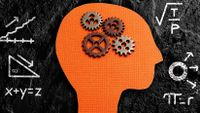George Lucas Educational Foundation
Teaching Strategies

# This Is What Math Looks Like

Whether they’re in kindergarten or calculus, students can use simulations to see the math.
September 13, 2017Math Simulations are tools I’ve created to visualize math ideas. They let students write math and see what it looks like by representing it visually through, for example, lengths, rotations, and light.

One simulation lets students write any number and see it as a length. Learners can type in the number 3, for instance, and see it presented as a line. Then they can type in the expression 5 x 3 and see that the line gets five times as long.

Another simulation allows students to represent a number of objects. Typing the number 3 displays 3 dots. Adding 5 makes 8 dots. Adding 5 more makes 13. Continuing to add 5 provides an intuitive way to visualize the function 3 + 5x.

This video shows what the simulations look like:

The simulations I’ve made can be combined to create functions (the simulations at the site are free, but registration is required). For instance, think about a dimmer switch for the lights in a room. You rotate the dimmer and the lights get brighter. There is a simulation that turns rotations into an amount of light. Just write any function, let’s say f(x) = 3x, and then turn the rotator in the simulation. The amount of light in the simulation will adjust to be three times the number of degrees of rotation.

Here’s another example. The length of a metal rod changes with its temperature. There’s a simulation for that: Write any function—for example, f(x) = 0.1arctan(x - 20) + 5—and then change the temperature in the simulation. The length will visually adjust according to the function.

This video highlights some functions that can be visualized with Math Simulations in the context of a playground:

### Know Activities, Do Activities, and Make Activities

Know activities: What I call Know activities help learners know the definitions of math concepts. They expose learners to examples of a concept as well as non-examples. Let’s take the concept of proportional relationships. A mechanical pencil can serve as a tangible example. Clicking the end of the pencil one time causes the lead to stick out 2 millimeters. Clicking a second time causes it to stick out 4 millimeters, and a third click makes it stick out 6 millimeters. This, we can explain to learners, is an example of a proportional relationship for two reasons: 1) When the number of clicks is zero, the length of the lead is zero; and 2) each click produces a constant increase in lead length: 2 millimeters.

Once the concept has been defined, Math Simulations allow us to produce many more visual examples quickly. For instance, we can open the temperature and time simulation and type the function y = 12x. Then slide the time slider in the simulator. Is the temperature zero when the time is zero? Yes. Does the temperature increase at a constant rate as the time increases? Yes. This is proportional. Non-examples can be produced just as easily. Typing f(x) = 50sin(x) will produce an example that clearly does not change at a constant rate.

Do activities: The second type of exercise, Do activities, helps learners translate between three representations of a concept: symbolic, real, and simulation. For instance, learners can be presented with a simulation and asked to write a symbolic function that models it. Or you can present them with a symbolic function and ask them to generate a real-world example of that function.

Make activities: Finally, Make activities help learners be creative with the math they learn. They ask learners to use mathematical simulations to imagine new possibilities in their world. In these activities, learners are presented with a real-world context along with a matching simulation and symbolic function. For example, they might be given the context of a fishing reel. After being cast, the reel is rotated to bring the line back in. The length of the fishing line is a function of the rotation of the reel. A function for this might be f(x) = 600 - (1/90)x, where x is in degrees and f(x) is in inches.

After familiarizing themselves with the situation, students are asked to write new functions that represent different fishing pole behaviors. These can range from slight adjustments to wild redesigns. The function f(x) = 700 - (1/90)x, for instance, implies a pole design with a longer fishing line. The function f(x) = 600 + 10sin(x) implies a design where rotating the reel pushes and pulls the line in and out. This is a potentially innovative fishing pole feature that might help lure fish, and it was imagined using pure mathematics.

### Final Thoughts

I’ve used Math Simulations with students in kindergarten through calculus, in classrooms where students have devices and ones where they don’t. In classes with one-to-one student devices, there’s a lot of potential for student creativity and ownership, but even when students don’t have devices, the simulations can still be useful, in whole class prompts and demonstrations.